This page lists all known errors in the book. Names in parentheses indicate who first spotted and reported the error. Thank you for sending any further bug reports to us via email.

### Correct version of Exercise 5.6 (p. 209) and its solution (p. 386)

Consider the two RDFS triples
r rdfs:domain A . and A rdfs:subClassOf B .
Understood as part of an OWL knowledge base, they can be expressed as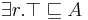$\exists r.\top \sqsubseteq A$ and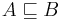$A \sqsubseteq B$.
Give a triple which is RDFS-entailed by the two given triples, but which cannot be derived from the OWL DL semantics.
Furthermore, give an OWL DL statement which is a logical consequence of the two OWL statements but cannot be derived using the RDFS semantics.

Solution:

The triple rdf:type rdfs:range rdfs:Class . is an axiomatic triple of RDFS and can thus be derived using the RDFS semantics. However, OWL DL does not allow us to use rdfs:Class; hence this statement cannot be derived using the OWL DL semantics.

The triple r rdfs:domain B . cannot be derived from the RDFS semantics. There is no easy way to check this, but you can exhaustively apply all deduction rules and then this triple will not be included. In DL syntax the triple becomes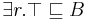$\exists r.\top \sqsubseteq B$, which is a logical consequence of the two given statements. To see this, let I be an arbitrarily chosen model for the two given statements. Then, using the definition of model and the two given statements, we obtain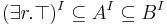$(\exists r.\top)^I \subseteq A^I \subseteq B^I$, which shows that$(\exists r.\top)^I \subseteq B^I$. Hence$\exists r.\top \sqsubseteq B$ holds in I, and since I was an arbitrarily chosen model,$\exists r.\top \sqsubseteq B$ holds in all models for the two given statements, and thus is a logical consequence from these two statements.

### Minor corrections and typos

• p. 2, footnote 1: "Dragonflies" should be "dragonflies"
• p. 11, heading of Section 1.4: "Semanic Web Technologies" should be "Semantic Web Technologies" (reported by Andreas Harth)
• p. 16, footnote 14: wrong font
• p. 27: "The overall RDF graph therefore consists of four edges and four nodes." should be "The overall RDF graph therefore consists of four edges and five nodes." (reported by Harald Zauner)
• p. 34, line 2: xml:namespace= should be xmlns:namespace=.
• p. 44, line 2: "alway" should be "always" (reported by Andrew Bate)
• p. 53, Figure 2.8: all occurrences of rdfs:resource should be replaced by rdf:resource (reported by Martin Wünsch)
• p. 54, second grey box: rdf:subPropertyOf should be rdfs:subPropertyOf. (reported by Tobias Käfer)
• p. 56, line 6: "rdfs:range" should be "rdfs:domain"
• p. 63, line above Section 2.5.2: this should read "( book:Hitzler book:Krötzsch book:Rudolph )" since the symbol "/" is not allowed here (reported by David Carral)
• p. 79, line 1: "Fig. 2.7" should be "Fig. 2.13" (reported by David Carral)
• p. 126, Figure 4.11: The last four lines of the OWL/RDF example should read: </owl:unionOf> </owl:Class> </rdfs:subClassOf> </owl:Class> (reported by Camille Pradel)
• p. 127, second OWL example: the class Person used on line 5 of the example should be replcaed by Professor to be consistent with the text on page 161 that refers to this example. (reported by Ignacio Lamata Martinez)
• p. 154, Section 4.4.1: owl:Ontology is missing from this list. (reported by Ron Koron)
• p. 181, last line: blank missing after comma. (reported by Manolis Koubarakis)
• p. 183, Section 5.3.1, item Class consistency: "To find out whether a given class D is inconsistent ..." should be "To find out whether a given class C is inconsistent ..." (reported by Heinz-Günter Kuper)
• p. 185, line 3: "NNF(K)" should be "NNF". (reported by Manolis Koubarakis)
• p. 193: In the example, x is not blocked by a1, since the latter is an individual. Refering to the tableau for the same example on p. 191, y is blocked by x, which suffices. (reported by Manolis Koubarakis)
• p. 194, line 19: "male" should be "Male"
• p. 196, line 6: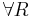$\forall R$ should only appear once in L(b). (reported by Manolis Koubarakis)
• p. 198, line 16 from below: The rightmost "Inv(R)" should simply be "R". (reported by Manolis Koubarakis)
• p. 201, line 3: the conjunction at the end of the line should be set-intersection. (reported by Manolis Koubarakis)
• p. 204, line 18: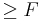$\geq F$ should be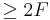$\geq 2 F$. (reported by Manolis Koubarakis)
• p. 204, line 24: replace "=" by "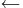$\leftarrow$. (reported by Manolis Koubarakis)
• p. 205, after step 8: Before step 9, the trans-rule needs to be applied to$\forall A.h\in \mathcal{L}(x_1)$. (reported by Manolis Koubarakis)
• p. 263: the example uses the URI http://semantic-web-book.org/uri/SemanticWeb for the book, which was denoted by http://semantic-web-book.org/uri/ in earlier examples. This should be unified, at least within this chapter. (reported by Frantisek Simancik)
• p. 269, line 5 in last paragraph: "A well-known example where this is not the case iw multiplication" should be "is" (reported by Birte Glimm)
• p. 340, line 21 "<foaf:knows>" is a duplicate of line 20 and should be deleted (reported by Dimitris Spanos)
• p. 354, last line, should end in encoding="utf-16"?>. This also occurs on pp. 356, 358, 359 (twice).
• p. 358, line 6 from below: type="xsd:string" should be type="&xsd;string". Similar errors occur later in Section A.3: wherever xsd: occurs in an attribute value, it should be replace by &xsd;.
• p. 361, last example: there are two occurrences of &xsd, which should be replaced by &xsd;.
• p. 371, bottom grey box: This should read "q(a) is a logical consequence of ... {p(a), ...}" (reported by George Planansky)
• p. 387, Exercise 5.7,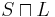$S\sqcap L$ occurring in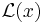$\mathcal{L}(x)$ should be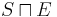$S\sqcap E$ (twice). (reported by Cong Wang)
• p. 410, reference [MKH08]: The authors, correctly stated, are M. Knorr, J. J. Alferes, and P. Hitzler.
• p. 424, main index entry for simplicity should be for page 169

Found an error – small or large – that is not listed on this page? Contact us now to make sure it will be gone in the next edition!